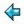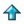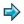### fibs.m

```fibs = map fib [0..]
fib 0 = 0
fib 1 = 1
fib (n+2) = fibs!(n+1) + fibs!n

test = layn (map shownum fibs)
```

This program tabulates the values of `fib i' a function for computing fibonacci numbers, in a list `fibs'. Because the function is memoised (i.e. it uses table lookup when it recurses) it runs in linear time. To see the fibonacci numbers say

```        test
```

There is a more direct way of defining fibs, using a list comprehension

```        fibs = [a | (a,b) <- (0,1), (b,a+b) ..]
```
this also runs in linear time